# C++内联函数详解

+关注继续查看

C++内联函数详解1.函数调用原理

"编译过程的最终产品是可执行程序--由一组机器语言指令组成。运行程序时，操作系统将这些指令载入计算机内存中，因此每条指令都有特定的内存地址。计算机随后将逐步执行这些指令。有时（如有循环和分支语句时），将跳过一些指令，向前或向后跳到特定地址。常规函数调用也使程序跳到另一个地址（函数的地址），并在函数结束时返回。下面更详细地介绍这一过程的典型实现。执行到函数调用指令时，程序将在函数调用后立即存储该指令的内存地址，并将函数参数复制到堆栈（为此保留的内存块），跳到标记函数起点的内存单元，执行函数代码（也许还需将返回值放入寄存器中），然后跳回到地址被保存的指令处（这与阅读文章时停下来看脚注，并在阅读完脚注后返回到以前阅读的地方类似）。来回跳跃并记录跳跃位置意味着以前使用函数时，需要一定的开销。"

2.内联函数

3.内联函数的使用

#include<iostream>
inline double square(double x){return x*x;}
int main()
{
using namespace std;
double a,b;
double c = 13.0;
a = square(5.0);
b = square(4.5+7.5);
cout<<"a="<<a<<",b="<<b<<endl;
cout<<"c="<<c<<endl;
cout<<"c squared="<<square(c++)<<endl;
cout<<"now c="<<c<<endl;
return 0;
}

a=25,b=144
c=13
c square=169
now c=14

4.内联函数与宏定义的区别
C语言使用预处理器语句#define来提供宏。如下例所示：
#define SQUARE(X) X*X

a = square(5.0);->a=5.0*5.0;
b = square(4.5+7.5);->b=4.5+7.5*4.5+7.5
d = square(c++);->d=c++*c++

#define SQUARE(X) ((X)*(X))

5.什么时候使用内联函数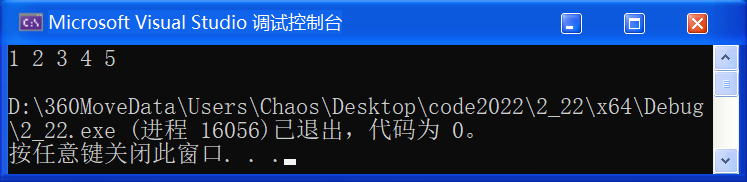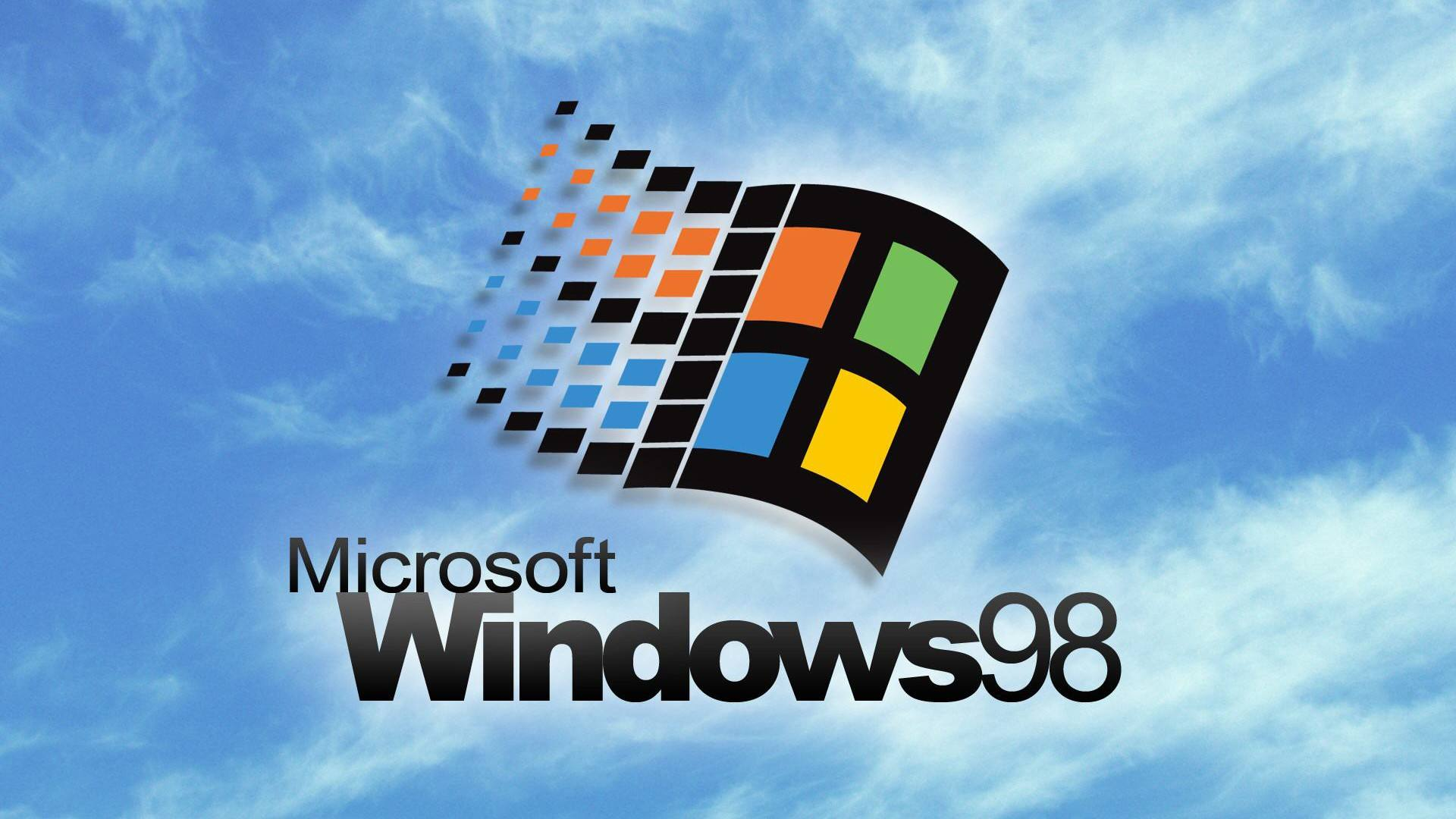【C++要笑着学】内联函数 inline | auto关键字（C++11） | 范围for | 关键字 nullptr（二）

0 0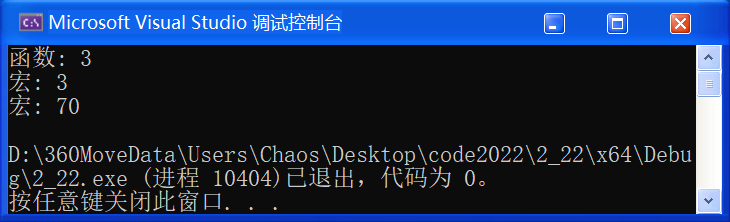【C++要笑着学】内联函数 inline | auto关键字（C++11） | 范围for | 关键字 nullptr（一）

0 0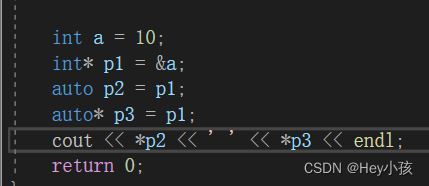C++程序设计——内联函数、auto、范围for循环、nullptr
C++程序设计——内联函数、auto、范围for循环、nullptr
0 0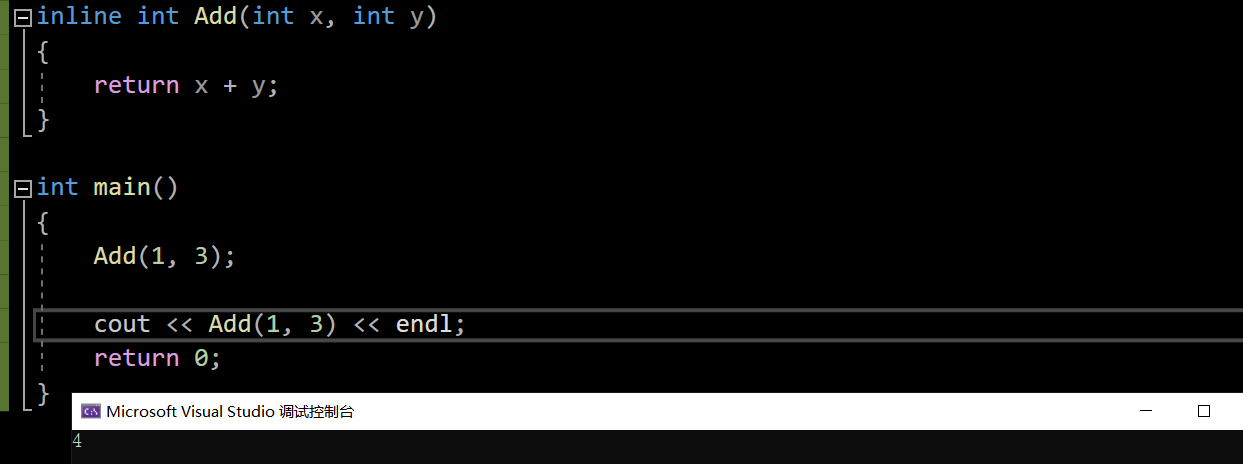C++——内联函数

0 0c++归来 内联函数

0 0

C++课程-对象模型

C++对象模型